Build a function generator with an Arduino Nano and a AD9833 Programmable Waveform Generator module.

IntermediateFull instructions provided12 hours1,302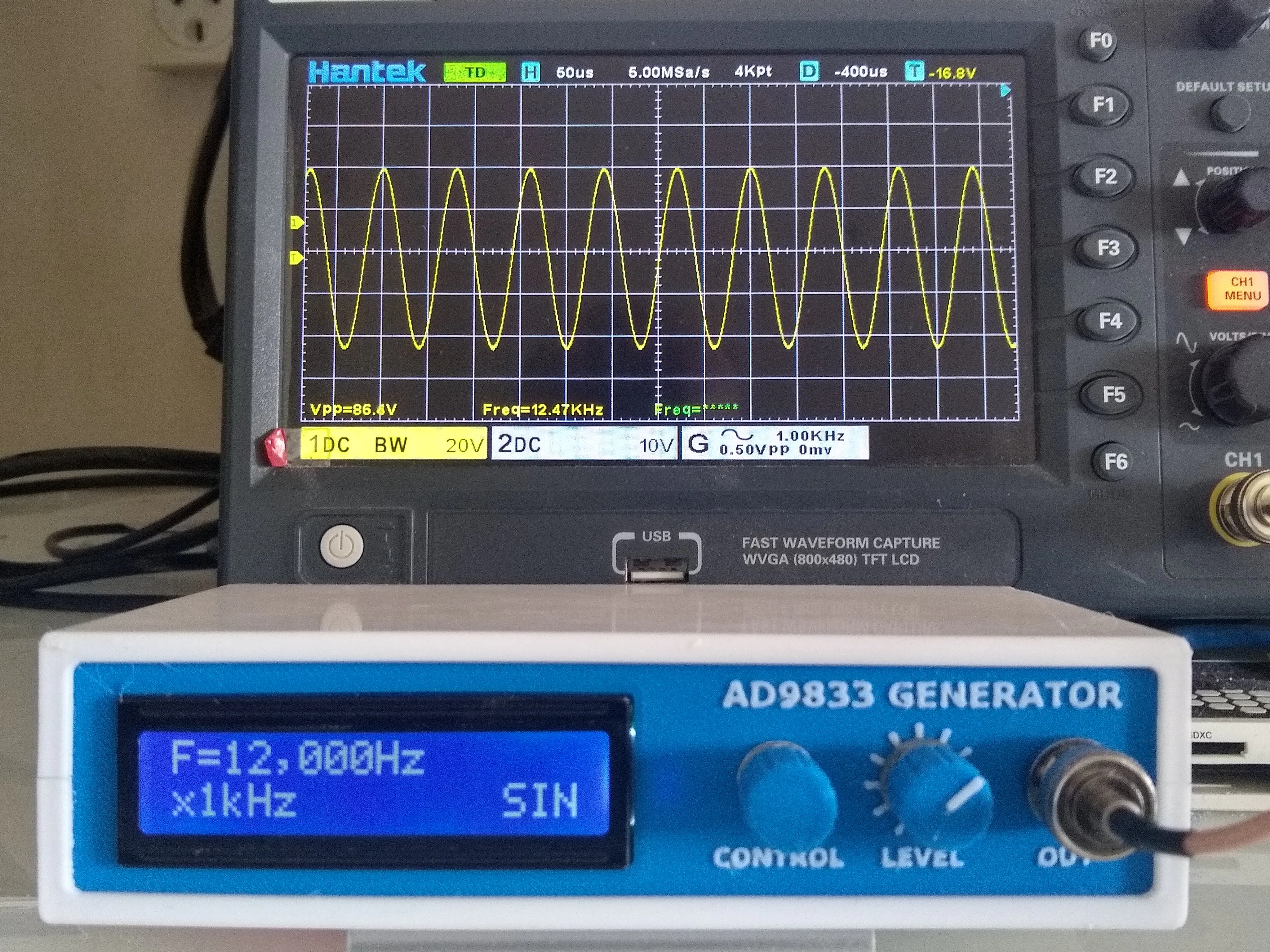## Things used in this project

### Hardware componentsArduino Nano R3
×1
×1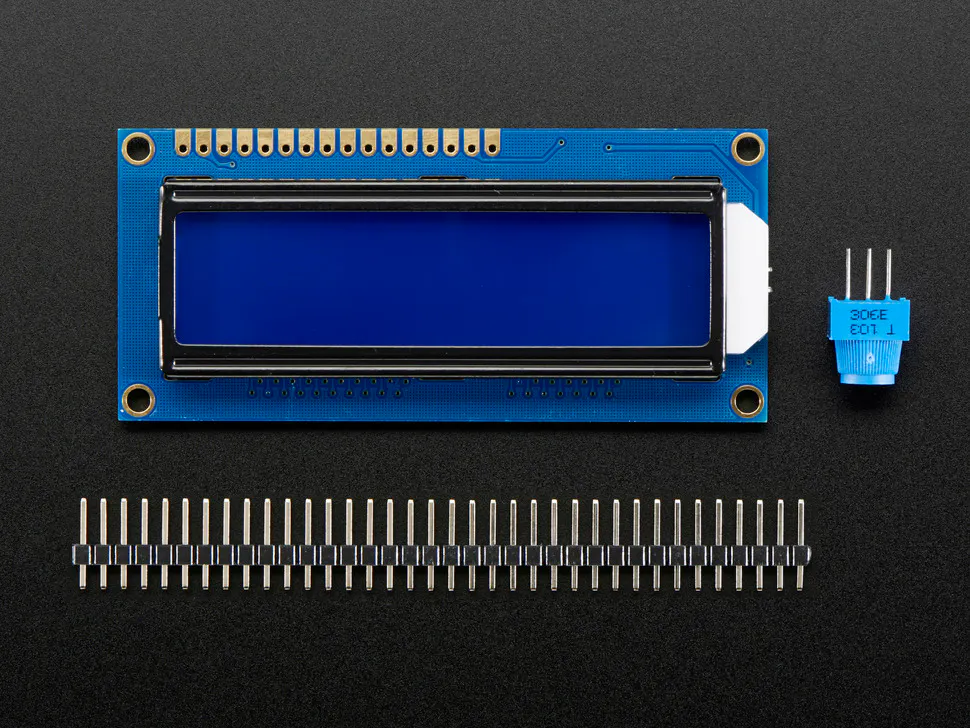Adafruit Standard LCD - 16x2 White on Blue
×1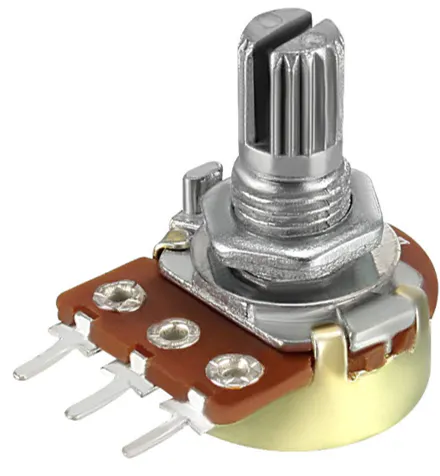DIYables Potentiometer 50k Linear
×1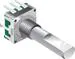Rotary Encoder with Push-Button 15mm D-Shaft
×1
 74HC14 SOIC-8 Package
×1
 TL071 DIL-8 Package
×1
 SparkFun 10k Trimpot
×1
 9V Battery holder
×2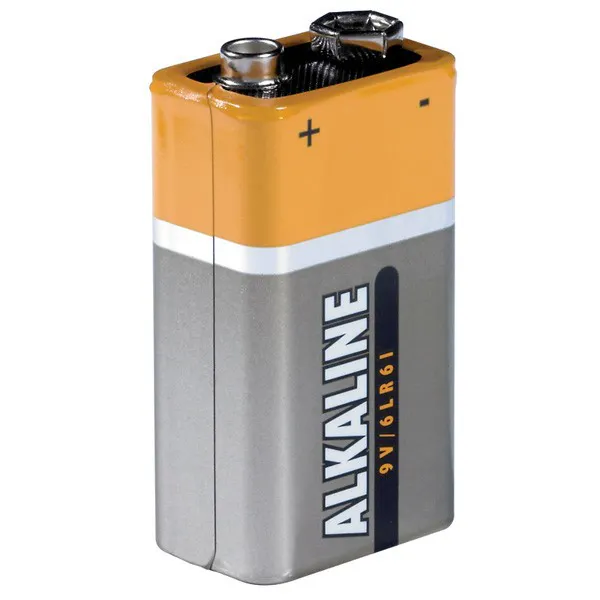9V battery (generic)
×2
 0805 Resistors 3 x 10k, 4 x 2.2k, 1 x 220R
×1
 0805 capacitors 3 x 22nF
×1

### Software apps and online servicesArduino IDE

### Hand tools and fabrication machines3D Printer (generic)Soldering iron (generic)

## Custom parts and enclosures

### STL Files

Files for 3D printing

## Schematics

### Schematic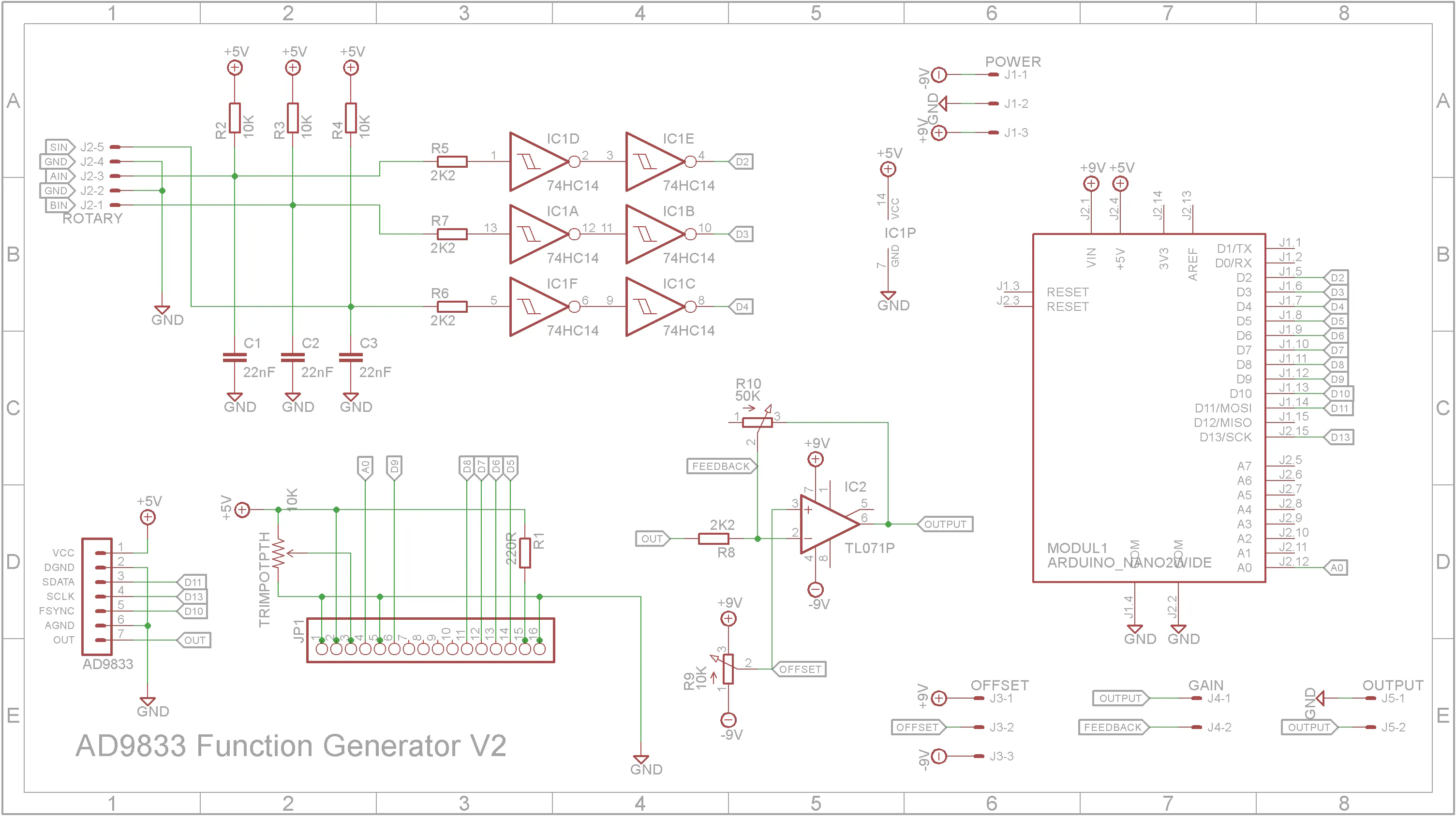### PCB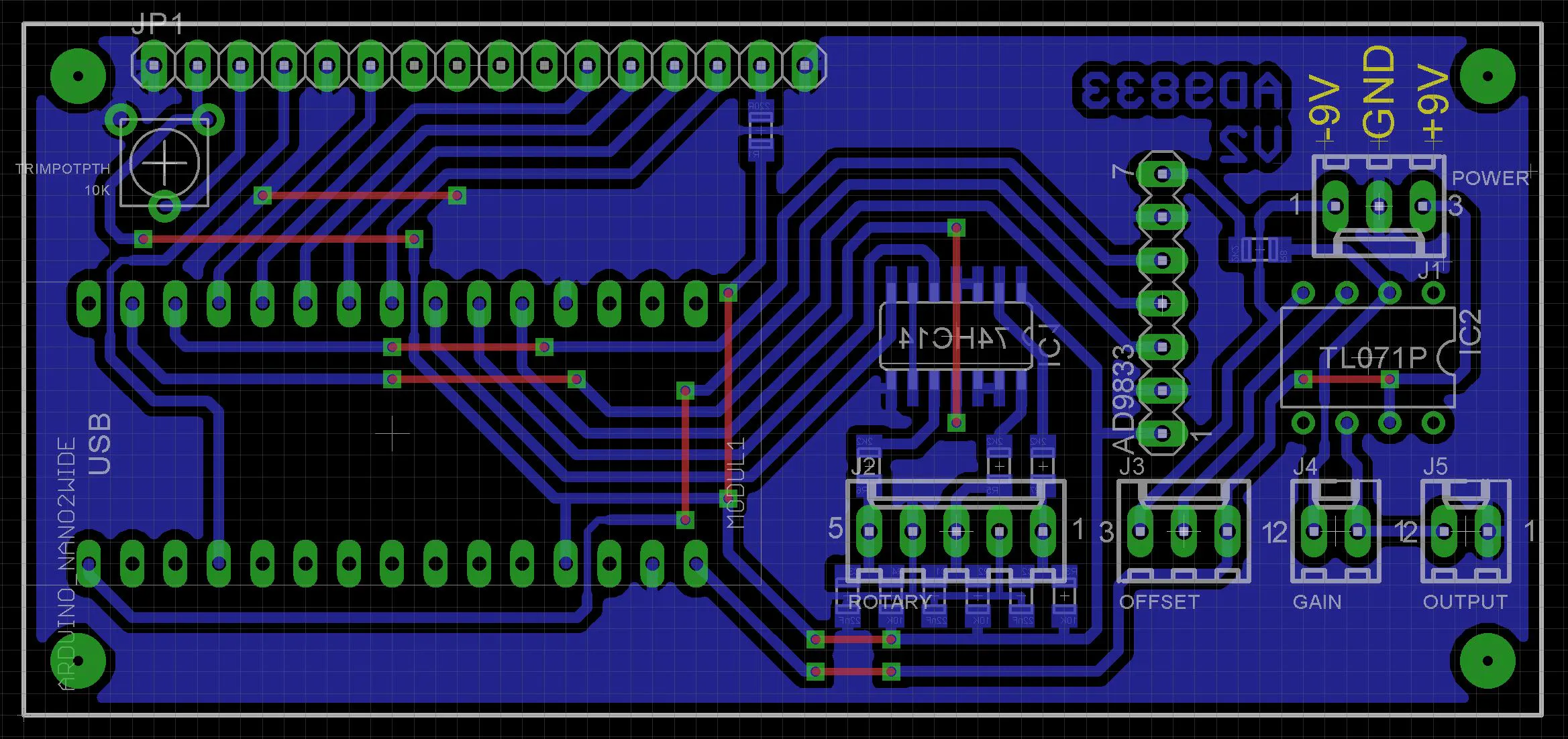### Eagle files

Schematic & PCB in Eagle format

## Code

### SignalGeneratorV3.ino

Arduino
```/*---------------------------------------------------------------------
* based on:
*  DIY Function/Waveform Generator by GreatScottLab
*  (https://www.instructables.com/DIY-FunctionWaveform-Generator/)
*
*  230114 - Rewrote code
*/
#include <LiquidCrystal.h>
#include <EEPROM.h>
#include "Rotary.h"

//Pins
#define ROTARY_A 3
#define ROTARY_B 2
#define SWITCH 4
#define LCD_D7 5
#define LCD_D6 6
#define LCD_D5 7
#define LCD_D4 8
#define LCD_EN 9
#define LCD_RS A0

//Modules
LiquidCrystal lcd(LCD_RS, LCD_EN, LCD_D4, LCD_D5, LCD_D6, LCD_D7);
Rotary encoder(ROTARY_A, ROTARY_B);	// Initialise the encoder on pins 2 and 3 (interrupt pins)

enum MenuEnum { X1, X10, X100, X1K, X10K, X100K, X1M, WAVEFORM };
enum WaveEnum { SINE, TRANGLE, SQUARE };
const String menuText[] = {"x1Hz", "x10Hz", "x100Hz","x1kHz", "x10kHz", "x100kHz", "x1MHz", "Waveform"};
const String waveText[] = {"SIN", "TRI", "SQR"};

//EEPROM handling
//Uncomment next line to clear out EEPROM and reset
//#define RESET_EEPROM
typedef struct {
uint32_t magic;
WaveEnum waveState;
long frequency;
} EEPROM_DATA;

EEPROM_DATA EepromData;      //Current EEPROM settings

#define EEPROM_UPDATE_TIME 60000  //Check for eprom update every minute
unsigned long eepromTimeout = 0;
bool eepromUpdate = false;

#define FREQ_MAX 14000000

volatile bool updateDisplay = false;

//--------------------------------------------------------------------
//Setup hardware
void setup()
{
//Serial.begin(57600);
//Serial.println("Starting...");

//Get last settings

// Initialise the LCD, start the backlight and print a "bootup" message for two seconds
lcd.begin(16, 2);
lcd.setCursor(0, 1);
lcd.print("Signal Generator");
delay(2000);

// Display initial set values
lcd.clear();
displayFrequency();
displayWaveform();

// Initialise the AD9833 with 1KHz sine output, no phase shift for both
// registers and remain on the FREQ0 register
// sigGen.lcdDebugInit(&lcd);
sigGen.reset(1);
sigGen.setFreq(EepromData.frequency);
sigGen.setPhase(0);
sigGen.setFPRegister(1);
sigGen.setFreq(EepromData.frequency);
sigGen.setPhase(0);
sigGen.setFPRegister(0);
sigGen.mode((int)EepromData.waveState);
sigGen.reset(0);

// Set pins A and B from encoder as interrupts
attachInterrupt(digitalPinToInterrupt(ROTARY_A), encChange, CHANGE);
attachInterrupt(digitalPinToInterrupt(ROTARY_B), encChange, CHANGE);
// Initialise pin as input with pull-up enabled and debounce variable for
// encoder button
pinMode(SWITCH, INPUT_PULLUP);
// Set Cursor to initial possition
lcd.setCursor(0, 1);

eepromTimeout = millis() + EEPROM_UPDATE_TIME;
}

//--------------------------------------------------------------------
//Main loop
void loop()
{
// If button is pressed, change the menu
if (testButton(true))
{
eepromUpdate = true;
updateDisplay = true;
}

// Update display if needed
if (updateDisplay)
{
displayFrequency();
displayWaveform();
updateDisplay = false;
}

//Update EEPROM if settings changed
if (millis() > eepromTimeout)
{
if (eepromUpdate)
{
writeEepromData();
eepromUpdate = false;
}
eepromTimeout = millis() + EEPROM_UPDATE_TIME;
}
}

//--------------------------------------------------------------------
// Test if button has been pressed
//  waitForRelease - True to wait until button gos up
//  Returns true if button is pressed
bool testButton(bool waitForRelease)
{
bool pressed = false;
{
pressed = true;
while (waitForRelease && digitalRead(SWITCH) == LOW)
{
yield();
}
}
return pressed;
}

//--------------------------------------------------------------------
// Encoder has been rotated
//  Change frequency or waveform based on menu selection
void encChange()
{
unsigned char state = encoder.process();
if (state != DIR_NONE)
{
{
case X1: updateFrequency(state,1); break;
case X10: updateFrequency(state,10); break;
case X100: updateFrequency(state,100); break;
case X1K: updateFrequency(state,1000); break;
case X10K: updateFrequency(state,10000); break;
case X100K: updateFrequency(state,100000); break;
case X1M: updateFrequency(state,1000000); break;
case WAVEFORM: updateWaveform(state); break;
}
}
}

//--------------------------------------------------------------------
//Change the current frequency based on menu and stepValue
//  state - Either DIR_CW or DIR_CCW
//  stepValue - Current amount to change frequency by
void updateFrequency(unsigned char state, long stepValue)
{
bool update = false;
long old = EepromData.frequency;
if (state == DIR_CW)
{
EepromData.frequency = min(EepromData.frequency + stepValue,FREQ_MAX);
}
else
{
EepromData.frequency = max(EepromData.frequency - stepValue,0);
}
if (old != EepromData.frequency)
{
sigGen.setFreq(EepromData.frequency);
eepromUpdate = true;
updateDisplay = true;
}
}

//--------------------------------------------------------------------
//Change the current waveform
//  state - Either DIR_CW or DIR_CCW
void updateWaveform(unsigned char state)
{
if (state == DIR_CW)
{
EepromData.waveState = (EepromData.waveState == SQUARE) ? SINE : (WaveEnum)((int)EepromData.waveState + 1);
}
else
{
EepromData.waveState = (EepromData.waveState == SINE) ? SQUARE : (WaveEnum)((int)EepromData.waveState - 1);
}
sigGen.mode((int)EepromData.waveState);
eepromUpdate = true;
updateDisplay = true;
}

//--------------------------------------------------------------------
//Display current frequency
void displayFrequency()
{
lcd.setCursor(0, 0);
lcd.print(formatNumber(EepromData.frequency,"F=","Hz",16));
}

//--------------------------------------------------------------------
{
lcd.setCursor(0, 1);
}

//--------------------------------------------------------------------
//Display active waveform
void displayWaveform()
{
lcd.setCursor(13, 1);
lcd.print(waveText[(int)EepromData.waveState]);
}

//-----------------------------------------------------------------------------------
//Display a number with comma seperators
// number - number to format
// prefix - String to prefix number with
// postfix - String to append to number
// returns String with formatted number
String formatNumber(long number, String prefix, String postfix, int pad)
{
String s = "";
bool space = true;
for (uint8_t i = 0; i < 8; i++)
{
if ((i==3 || i==6) && !space && number > 0)
{
s = String(',') + s;
}
if (number > 0 || i == 0)
{
s = String((char)((number % 10) + 48)) + s;
space = false;
}
else
{
space = true;
}
number = number / 10;
}
s = prefix + s + postfix;
}

//-----------------------------------------------------------------------------------
// s - string to pad
// prefix - String to prefix number with
{
String sOut = String(s);
int len = pad - s.length();
for (uint8_t i = 0; i < len; i++)
{
sOut += ' ';
}
return sOut;
}

//--------------------------------------------------------------------
//Write the EepromData structure to EEPROM
void writeEepromData(void)
{
//This function uses EEPROM.update() to perform the write, so does not rewrites the value if it didn't change.
}

//--------------------------------------------------------------------
//Read the EepromData structure from EEPROM, initialise if necessary
{
#ifndef RESET_EEPROM
if (EepromData.magic != EEPROM_MAGIC)
{
#endif
EepromData.magic = EEPROM_MAGIC;
EepromData.waveState = SINE;
EepromData.frequency = 1000;
writeEepromData();
#ifndef RESET_EEPROM
}
#endif
}
```

```#ifndef AD9833_H

#include <SPI.h>
//#include "LiquidCrystal_I2C.h"

{
public:
//_FYNC is the pin on the uC where FYNC is connected
//_mclk is the frequency of the crystal generator
//Set and get for frequency
void setFreq(unsigned long _freq);
unsigned long getFreq();
//Set and get for phase
void setPhase(int _phase);
int getPhase();
//Set and get for control register
void setCtrlReg(unsigned long _controlRegister);
int getCtrlReg();
void writeData(int data);
//Send the control register
void writeCtrlReg();
//Update the control register with appopiate values for sleep/reset/mode
//s = 0 No power down
//s = 1 DAC powered down
//s = 2 Internal clock powered down
//s = 3 Both DAC and internal clock powered down
void sleep(int s);
//r = 0 Disable Reset
//r = 1 Enable Reset
void reset(int r);
//m = 0 Sine
//m = 1 Triangle
//m = 2 Clock (rectangle)
void mode(int m);
//r = 0 FREQ0 and PHASE0 are used
//r = 1 FREQ1 and PHASE1 are used
void setFPRegister(int r);
//void lcdDebugInit(LiquidCrystal_I2C *);
//void lcdDebug(String);

private:
int FSYNC;          //FSYNC Pin of AD9833 has to be connected to a GPIO pin
unsigned long freq; //Output frequency
int phase;          //Signal phase shift
unsigned long mclk; //External oscillator frequency
int fqRegister;    //Switch between Frequency and Phase register 1/0
int pRegister;
int controlRegister;
//LiquidCrystal_I2C *debugger; //Debugging stuff through I2C
//boolean debugging;
};

#endif
```

C/C++
```#include <SPI.h>

FSYNC = _FSYNC;
pinMode(FSYNC, OUTPUT);     // Set FSYNC pin as input
mclk = _mclk;               // Set the oscillator used, for example 24MHz
controlRegister = 0x2000;   // Default control register : FREQ0, PHASE0, Sine
fqRegister = 0x4000;        // Default frequency register is 0
pRegister = 0xC000;         // Default phase register is 0
SPI.begin();                // Initialise the SPI BUS
SPI.setDataMode(SPI_MODE2); // Set SPI in mode2, this should be moved in
// methods when SPI.transfer is called in case you
// have multiple devices using the SPI bus
}

// FSYNC pin must be pulled low when new data is received by AD9833
digitalWrite(FSYNC, LOW);
// Send the first 8 MSBs of data
SPI.transfer(highByte(data));
// Send the last 8 LSBs of data
SPI.transfer(lowByte(data));
// Set the FSYNC pin to high then end SPI transaction
digitalWrite(FSYNC, HIGH);
// debugging
/*
if (debugging) {
lcdDebug(String(data, HEX));
}
*/
}

// First check that the data received is fine
unsigned long freqReg;
// Frequency cannot be negative
if (_freq < 0) {
freqReg = 0;
freq = 0;
}
// If the frequency is more than maximum frequency, just set it to maximum
else if (_freq > mclk) {
freqReg = pow(2, 28) - 1;
freq = mclk / 2;
}
// If all is good, compute the freqReg knowhing that the analog output is
// (mclk/2^28) * freqReg
else {
freq = _freq;
freqReg = (freq * pow(2, 28)) / mclk;
}
// Initialise two variables that are 16bit long which we use to divide the
// freqReg in two words
// set D15 to 0 and D14 to 1 to put data in FREQ0/1 register
int MSW = ((int)(freqReg >> 14)) | fqRegister; // Take out the first 14bits
// and set D15 to 0 and D14 to
// 1 or viceversa depending on
// FREQ reg
int LSW = ((int)(freqReg & 0x3FFF)) |
fqRegister; // Take only the last 14bits using a mask and set D15 to
// 0 and D14 to 1 or viceversa depending on FREQ reg
// Send the data, most significant word first
writeData(LSW);
writeData(MSW);
}
return freq;
}
// Phase cannot be negative
if (_phase < 0) {
phase = 0;
}
// Phase maximum is 2^12
else if (_phase >= 4096) {
phase = 4096 - 1;
}
// If all is good, set the new phase value
else {
phase = _phase;
}
// Extract the 12 bits from the freqReg and set D15-1, D14-1, D13-0, D12-X to
// put data in PHASE0/1 register
int phaseData = phase | pRegister;
int LSW = (phase & 0x3FFF) | pRegister;
writeData(phaseData);
}
return phase;
}

// Just make sure that the first two bits are set to 0
controlRegister = _controlRegister & 0x3FFF;
writeCtrlReg();
}

return controlRegister;
}

writeData(controlRegister);
}

switch (s) {

case 0: {
controlRegister &= 0xFF3F; // No power-down: D7-0 and D6-0
} break;

case 1: {
controlRegister &= 0xFF7F; // DAC powered down: D7-0 and D6-1
controlRegister |= 0x0040;
} break;

case 2: {
controlRegister &= 0xFFBF; // Internal clock disabled: D7-1 and D6-0
controlRegister |= 0x0080;
} break;

case 3: {
controlRegister |=
0x00C0; // Both DAC powered down and internal clock disabled
} break;
}
// Update the control register
writeCtrlReg();
}

if (r == 0) {
controlRegister &= 0xFEFF; // Set D8 to 0
}

else if (r == 1) {
controlRegister |= 0x0100; // Set D8 to 1
}
writeCtrlReg();
}

switch (m) {
case 0: {
controlRegister &= 0xFFDD; // Output sine: D5-0 and D1-0
} break;
case 1: {
controlRegister &= 0xFFDF; // Output triangle: D5-0 and D1-1
controlRegister |= 0x0002;
} break;
case 2: {
controlRegister &= 0xFFFD; // Output clock (rectangle): D5-1 and D1-0
controlRegister |= 0x0020;
} break;
}
writeCtrlReg();
}

if (r == 0) {
controlRegister &= 0xF3FF; // Set D11 and D10 in control register to 0
fqRegister = 0x4000; // Set D15 to 0 and D14 to 1 in a variable that will
// later choose the FREQ0 register
pRegister =
0xC000; // Set D15 to 1 and D14 to 1 and D13 to 0 for the PHASE register
} else if (r == 1) {
controlRegister |= 0x0C00; // Set D11 and D10 in control register to 1
fqRegister = 0x8000; // Set D15 to 1 and D14 to 0 in a variable that will
// later choose the FREQ1 register
pRegister =
0xD000; // Set D15 to 1 and D14 to 1 and D13 to 1 for the PHASE register
}
writeCtrlReg();
}

/*
debugger = _debugger;
debugging = true;
}

if (debugging) {
debugger->setCursor(0, 1);
debugger->print(message);
}
}
*/
```

### Rotary.h

```/*
* Rotary encoder library for Arduino.
*/

#ifndef rotary_h
#define rotary_h

#include "Arduino.h"

// Enable this to emit codes twice per step.
//#define HALF_STEP

// Enable weak pullups
#define ENABLE_PULLUPS

// Values returned by 'process'
// No complete step yet.
#define DIR_NONE 0x0
// Clockwise step.
#define DIR_CW 0x10
// Anti-clockwise step.
#define DIR_CCW 0x20

class Rotary
{
public:
Rotary(char, char);
// Process pin(s)
unsigned char process();
private:
unsigned char state;
unsigned char pin1;
unsigned char pin2;
};

#endif

```

### Rotary.cpp

C/C++
```/* Rotary encoder handler for arduino. v1.1
*
* Copyright 2011 Ben Buxton. Licenced under the GNU GPL Version 3.
* Contact: bb@cactii.net
*
* A typical mechanical rotary encoder emits a two bit gray code
* on 3 output pins. Every step in the output (often accompanied
* by a physical 'click') generates a specific sequence of output
* codes on the pins.
*
* There are 3 pins used for the rotary encoding - one common and
* two 'bit' pins.
*
* The following is the typical sequence of code on the output when
* moving from one step to the next:
*
*   Position   Bit1   Bit2
*   ----------------------
*     Step1     0      0
*      1/4      1      0
*      1/2      1      1
*      3/4      0      1
*     Step2     0      0
*
* From this table, we can see that when moving from one 'click' to
* the next, there are 4 changes in the output code.
*
* - From an initial 0 - 0, Bit1 goes high, Bit0 stays low.
* - Then both bits are high, halfway through the step.
* - Then Bit1 goes low, but Bit2 stays high.
* - Finally at the end of the step, both bits return to 0.
*
* Detecting the direction is easy - the table simply goes in the other
*
* To decode this, we use a simple state machine. Every time the output
* code changes, it follows state, until finally a full steps worth of
* code is received (in the correct order). At the final 0-0, it returns
* a value indicating a step in one direction or the other.
*
* It's also possible to use 'half-step' mode. This just emits an event
* at both the 0-0 and 1-1 positions. This might be useful for some
* encoders where you want to detect all positions.
*
* If an invalid state happens (for example we go from '0-1' straight
* to '1-0'), the state machine resets to the start until 0-0 and the
* next valid codes occur.
*
* The biggest advantage of using a state machine over other algorithms
* is that this has inherent debounce built in. Other algorithms emit spurious
* output with switch bounce, but this one will simply flip between
* sub-states until the bounce settles, then continue along the state
* machine.
* A side effect of debounce is that fast rotations can cause steps to
* be skipped. By not requiring debounce, fast rotations can be accurately
* measured.
* Another advantage is the ability to properly handle bad state, such
* as due to EMI, etc.
* It is also a lot simpler than others - a static state table and less
* than 10 lines of logic.
*/

#include "Arduino.h"
#include "Rotary.h"

/*
* The below state table has, for each state (row), the new state
* to set based on the next encoder output. From left to right in,
* the table, the encoder outputs are 00, 01, 10, 11, and the value
* in that position is the new state to set.
*/

#define R_START 0x0

#ifdef HALF_STEP
// Use the half-step state table (emits a code at 00 and 11)
#define R_CCW_BEGIN 0x1
#define R_CW_BEGIN 0x2
#define R_START_M 0x3
#define R_CW_BEGIN_M 0x4
#define R_CCW_BEGIN_M 0x5
const unsigned char ttable = {
// R_START (00)
{R_START_M,            R_CW_BEGIN,     R_CCW_BEGIN,  R_START},
// R_CCW_BEGIN
{R_START_M | DIR_CCW, R_START,        R_CCW_BEGIN,  R_START},
// R_CW_BEGIN
{R_START_M | DIR_CW,  R_CW_BEGIN,     R_START,      R_START},
// R_START_M (11)
{R_START_M,            R_CCW_BEGIN_M,  R_CW_BEGIN_M, R_START},
// R_CW_BEGIN_M
{R_START_M,            R_START_M,      R_CW_BEGIN_M, R_START | DIR_CW},
// R_CCW_BEGIN_M
{R_START_M,            R_CCW_BEGIN_M,  R_START_M,    R_START | DIR_CCW},
};
#else
// Use the full-step state table (emits a code at 00 only)
#define R_CW_FINAL 0x1
#define R_CW_BEGIN 0x2
#define R_CW_NEXT 0x3
#define R_CCW_BEGIN 0x4
#define R_CCW_FINAL 0x5
#define R_CCW_NEXT 0x6

const unsigned char ttable = {
// R_START
{R_START,    R_CW_BEGIN,  R_CCW_BEGIN, R_START},
// R_CW_FINAL
{R_CW_NEXT,  R_START,     R_CW_FINAL,  R_START | DIR_CW},
// R_CW_BEGIN
{R_CW_NEXT,  R_CW_BEGIN,  R_START,     R_START},
// R_CW_NEXT
{R_CW_NEXT,  R_CW_BEGIN,  R_CW_FINAL,  R_START},
// R_CCW_BEGIN
{R_CCW_NEXT, R_START,     R_CCW_BEGIN, R_START},
// R_CCW_FINAL
{R_CCW_NEXT, R_CCW_FINAL, R_START,     R_START | DIR_CCW},
// R_CCW_NEXT
{R_CCW_NEXT, R_CCW_FINAL, R_CCW_BEGIN, R_START},
};
#endif

/*
* Constructor. Each arg is the pin number for each encoder contact.
*/
Rotary::Rotary(char _pin1, char _pin2) {
// Assign variables.
pin1 = _pin1;
pin2 = _pin2;
// Set pins to input.
pinMode(pin1, INPUT);
pinMode(pin2, INPUT);
#ifdef ENABLE_PULLUPS
digitalWrite(pin1, HIGH);
digitalWrite(pin2, HIGH);
#endif
// Initialise state.
state = R_START;
}

unsigned char Rotary::process() {
// Grab state of input pins.
// Determine new state from the pins and state table.
state = ttable[state & 0xf][pinstate];
// Return emit bits, ie the generated event.
return state & 0x30;
}
```